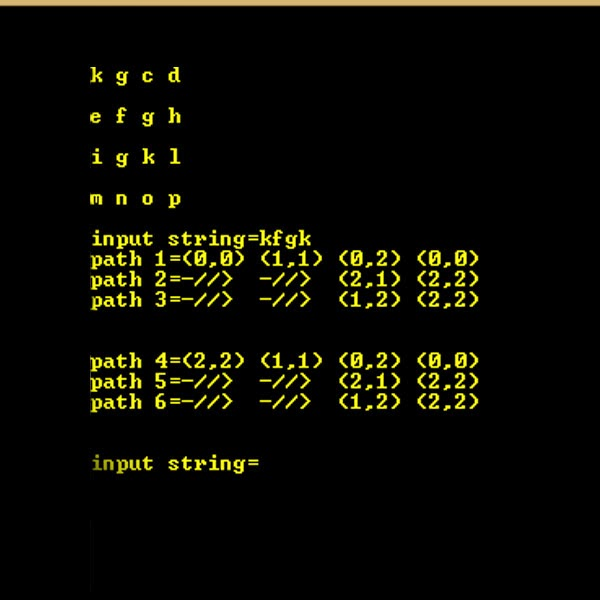## Posts

### Segment Tree

Segment Tree | Set 1 (Sum of given range) Let us consider the following problem to understand Segment Trees. We have an array arr[0 . . . n-1]. We should be able to 1 Find the sum of elements from index l to r where 0 <= l <= r <= n-1 2 Change value of a specified element of the array arr[i] = x where 0 <= i <= n-1.
A girl counting on her left hand’s fingers, u need to tell on which finger she will stop for a giving number. Counting was like 1-thumb, 2-index finger , 3-middle finger, 4-ring finger,5-little finger then 6-ring, 7-middle , 8-index, 9 thumb , 10 -index and so on
Splendid Matrices Splendid Matrices C++ Program Rani has challenged Nandu to generate Splendid Matrices. Splendid matrices are square matrices with dimensions 2n X 2n filled in a particular manner. To explain this manner, Rani gives Nandu the matrices for n=1, n=2 and n=3 :

### Trie |(Insert and Search )

Trie | (Insert and Search) Trie is an efficient information retrieval data structure. Using trie, search complexities can be brought to optimal limit (key length). If we store keys in binary search tree, a well balanced BST will need time proportional to M * log N, where M is maximum string length and N is number of keys in tree. Using trie, we can search the key in O(M) time. However the penalty is on trie storage requirements.
Every node of trie consists of multiple branches. Each branch represents a possible character of keys. We need to mark the last node of every key as leaf node. A trie node field value will be used to distinguish the node as leaf node (there are other uses of the value field). A simple structure to represent nodes of English alphabet can be as following,
structtrie_node { intvalue; /* Used to mark leaf nodes */ trie_node_t *children[ALPHABET_SIZE]; }; Inserting a key into trie is simple approach. Every character of input key is inserted as an individual trie n…

### WORDAMENT( WAP to search a word in N*N grid with one char in each cell.)eg......

keep coding....... work hard have fun make history @hios